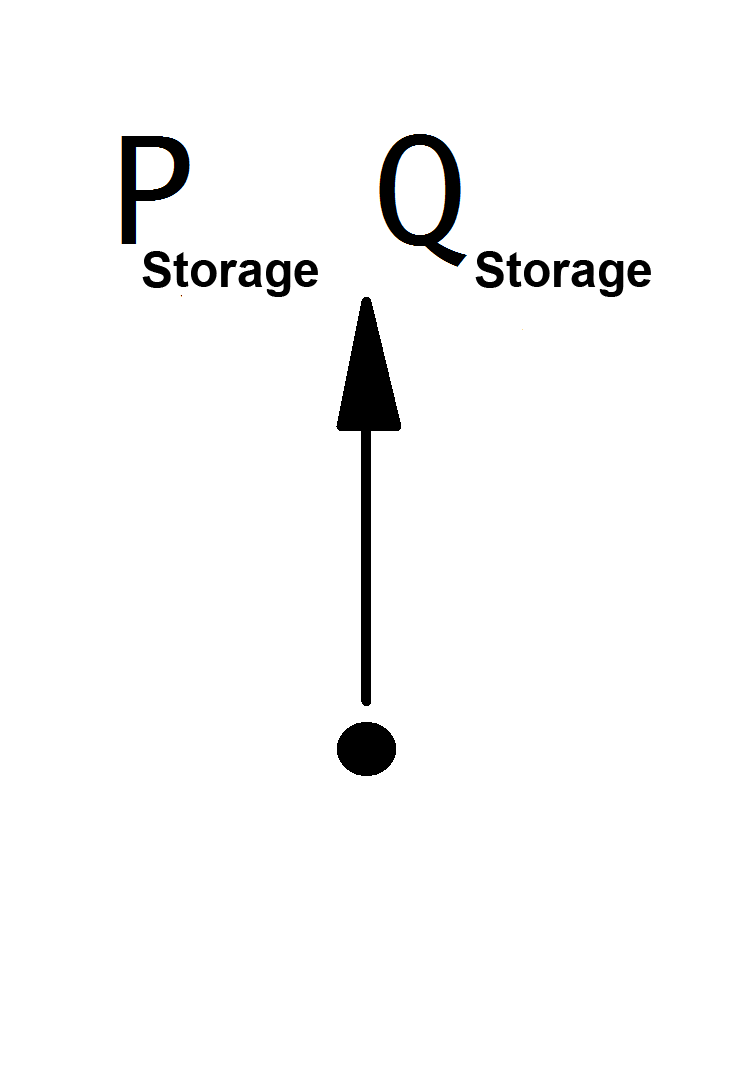# Storage¶

Note

Since storage power values are given in the consumer system, positive power models charging and negative power models discharging.

## Create Function¶

pandapower.create_storage(net, bus, p_mw, max_e_mwh, q_mvar=0, sn_mva=nan, soc_percent=nan, min_e_mwh=0.0, name=None, index=None, scaling=1.0, type=None, in_service=True, max_p_mw=nan, min_p_mw=nan, max_q_mvar=nan, min_q_mvar=nan, controllable=nan)

Adds a storage to the network.

In order to simulate a storage system it is possible to use sgens or loads to model the discharging or charging state. The power of a storage can be positive or negative, so the use of either a sgen or a load is (per definition of the elements) not correct. To overcome this issue, a storage element can be created.

As pandapower is not a time dependend simulation tool and there is no time domain parameter in default power flow calculations, the state of charge (SOC) is not updated during any power flow calculation. The implementation of energy content related parameters in the storage element allows to create customized, time dependend simulations by running several power flow calculations and updating variables manually.

INPUT:

net - The net within this storage should be created

bus (int) - The bus id to which the storage is connected

p_mw (float) - The momentary real power of the storage (positive for charging, negative for discharging)

max_e_mwh (float) - The maximum energy content of the storage (maximum charge level)

OPTIONAL:

q_mvar (float, default 0) - The reactive power of the storage

sn_mva (float, default None) - Nominal power of the storage

soc_percent (float, NaN) - The state of charge of the storage

min_e_mwh (float, 0) - The minimum energy content of the storage (minimum charge level)

name (string, default None) - The name for this storage

index (int, None) - Force a specified ID if it is available. If None, the index one higher than the highest already existing index is selected.

scaling (float, 1.) - An OPTIONAL scaling factor to be set customly

type (string, None) - type variable to classify the storage

in_service (boolean) - True for in_service or False for out of service

max_p_mw (float, NaN) - Maximum active power injection - necessary for a controllable storage in OPF

min_p_mw (float, NaN) - Minimum active power injection - necessary for a controllable storage in OPF

max_q_mvar (float, NaN) - Maximum reactive power injection - necessary for a controllable storage in OPF

min_q_mvar (float, NaN) - Minimum reactive power injection - necessary for a controllable storage in OPF

controllable (bool, NaN) - Whether this storage is controllable by the optimal powerflow

OUTPUT:

index (int) - The unique ID of the created storage

EXAMPLE:

create_storage(net, 1, p_mw = -30, max_e_mwh = 60, soc_percent = 1.0, min_e_mwh = 5)

## Input Parameters¶

net.storage

 Parameter Datatype Value Range Explanation name string Name of the storage unit bus* integer Index of connected bus p_mw* float $$\leq$$ 0 Momentary real power of the storage (positive for charging, negative for discharging) q_mvar* float Reactive power of the storage [MVar] sn_mva float $$>$$ 0 Nominal power ot the storage [MVA] scaling* float $$\geq$$ 0 Scaling factor for the active and reactive power max_e_mwh float The maximum energy content of the storage (maximum charge level) min_e_mwh float The minimum energy content of the storage (minimum charge level) max_p_mw** float Maximum active power min_p_mw** float Minimum active power soc_percent float 0 $$\leq$$ soc_percent $$\leq$$ 100 The state of charge of the storage max_q_mvar** float Maximum reactive power [MVar] min_q_mvar** float Minimum reactive power [MVar] controllable** bool States if sgen is controllable or not, sgen will not be used as a flexibilty if it is not controllable in_service* boolean True / False Specifies if the generator is in service

*necessary for executing a power flow calculation
**optimal power flow parameter

## Electric Model¶

Storages are modelled as PQ-buses in the power flow calculation:The PQ-Values are calculated from the parameter table values as:

\begin{align*} P_{storage} &= p\_mw \cdot scaling \\ Q_{storage} &= q\_mvar \cdot scaling \\ \end{align*}

Note

The apparent power value sn_mva, state of charge soc and storage capacity max_e_mwh are provided as additional information for usage in controller or other applications based on panadapower. It is not considered in the power flow!

## Result Parameters¶

net.res_storage

 Parameter Datatype Explanation p_mw float resulting active power after scaling [MW] q_mvar float resulting reactive power after scaling [MVar]

The power values in the net.res_storage table are equivalent to $$P_{storage}$$ and $$Q_{storage}$$.